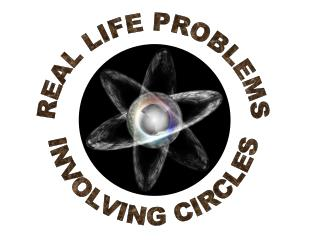Download PresentationREAL LIFE PROBLEMS INVOLVING CIRCLES

REAL LIFE PROBLEMS INVOLVING CIRCLES - PowerPoint PPT Presentation

REAL LIFE PROBLEMS INVOLVING CIRCLES. C. D. 3 cm. 3 cm. G. H. A. 12 cm. B. Length of Belt = m CD + m DHF + m FE + m EGC. E. F. m CD = m FE = 12 cm. m DHF = m EGC = one-half of the circumference.I am the owner, or an agent authorized to act on behalf of the owner, of the copyrighted work described.
Download PresentationREAL LIFE PROBLEMS INVOLVING CIRCLES

Download Policy: Content on the Website is provided to you AS IS for your information and personal use and may not be sold / licensed / shared on other websites without getting consent from its author.While downloading, if for some reason you are not able to download a presentation, the publisher may have deleted the file from their server.

- - - - - - - - - - - - - - - - - - - - - - - - - - E N D - - - - - - - - - - - - - - - - - - - - - - - - - -
Presentation Transcript
1. REAL LIFE PROBLEMS INVOLVING CIRCLES

2. C D 3 cm 3 cm G H A 12 cm B Length of Belt = m CD + m DHF + m FE+ m EGC E F m CD = m FE = 12 cm m DHF = m EGC = one-half of the circumference There are two wheels in a washing machine each with a radius of 3 cm. The centres of those wheels are separated by 12 cm. The two wheels are connected by a belt around them as shown. How long is this belt? To determine the length of the belt we will divide the belt into 4 sections. = = r = 3.14(3) =9.42 cm Length of Belt = 12 + 9.42 +12 + 9.42 = 42.84 cm

3. B S mASB = 46 V P T A mASB = m APB -m AVB 2 Let m AVB = x Percentage = = 37.2%  m APB = 360 - x Satellite S receives signals from Control Station A and retransmits them to Control Station B. All cities located on arc AVB can receive the signals from the satellite. Given that mASB = 46, what percentage of the Earth’s circumference will receive the signals? 92 = 360 - 2x 2x = 360 - 92 2x = 268 x = 134

4. Martha and Paul bought their cars at the same time and they drive under similar conditions, except that Martha works farther away from home than Paul. When they had their tires checked at a garage, they found out that their tires were equally worn, even though Martha had driven 36923 km and Paul only 32000km. If the diameter of Paul’s wheel is 32.5 cm, what is that of Martha’s. On each tire rotation the car travels one circumference. This means that a car with bigger tires can travel farther on one rotation. Also the distance a car travels depends on the number of rotations of the tire and the circumference of the tire. Distance travelled = number of rotations  Circumference x = n  C

5. Distance travelled by Paul Distance travelled by Martha xP = 32000 km = nP CP xM = 36923 km = nM CM The amount of tire wear depends on the number of rotations that a tire makes. If a large tire is worn down the same amount as a smaller tire, then they both had the same number of rotations.  nP= nM According to Theorem 11 Since nP= nM 32000dM= (32.5 cm)(36923) dM = 37.5 cm

6. 30 cm 20 cm or Pete’s Pie Palace is going to be open for business in a few weeks. In fact, Henry the baker is busy preparing the menu and setting the prices. Naturally, he wants the prices to be proportional to the size of the pies. After doing a little cost analysis, he decides that a pie with a diameter of 20 cm will cost \$6.00. What should a pie with a diameter of 30 cm cost? The cost of a pie should be proportional to the amount of food (i.e. – the area of the pie)  Ratio of the cost of pies = Ratio of the area of pies and Th. 12 \$6.00 \$ x  x = \$13.50 4x = 54

7. That's a Wrap